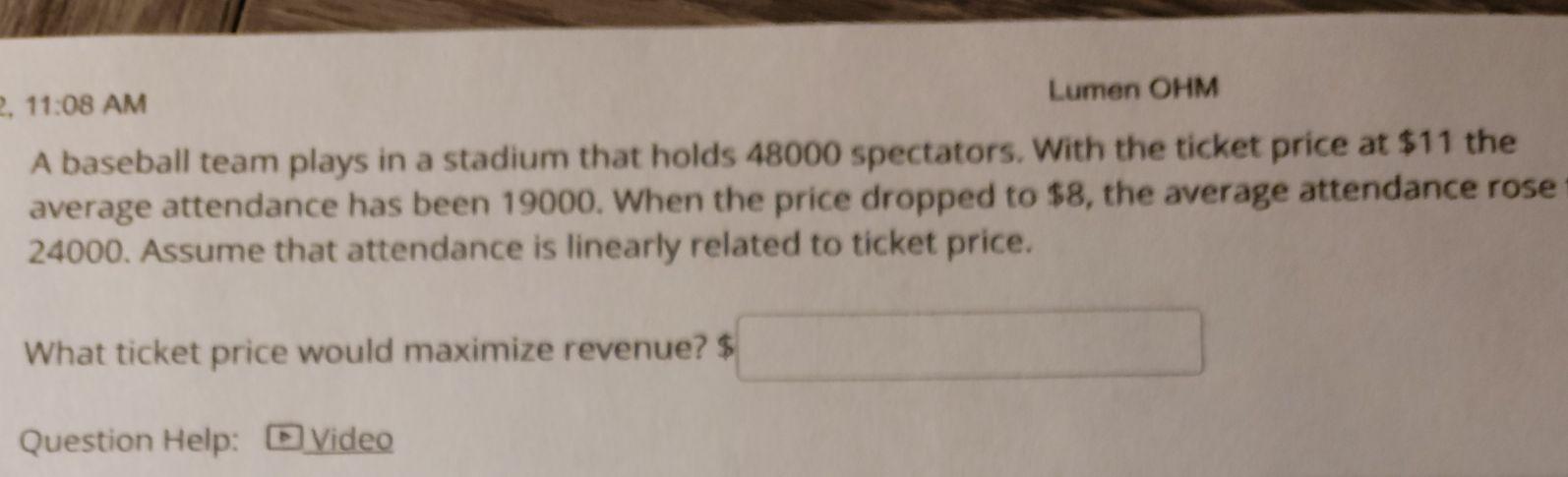### ¿Todavía tienes preguntas de matemáticas?

Pregunte a nuestros tutores expertos
Algebra
PreguntaA baseball team plays in a stadium that holds $$48000$$ spectators. With the ticket price at $$\ 11$$ the average attendance has been $$19000$$ . When the price dropped to $$\ 8$$ , the average attendance rose $$24000$$ . Assume that attendance is linearly related to ticket price.

What ticket price would maximize revenue? $$\$$ ____